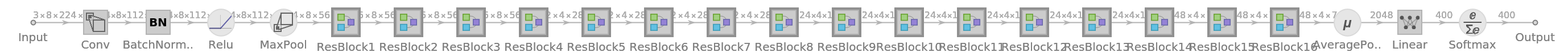# 3D-Inflated ResNet-50 Trained onKinetics 400 Data

Identify the main action in a video

This model applies a 3D-inflation technique to bootstrap the kernels of a 3D convolutional network from a 2D ResNet-50 architecture, directly leveraging years of progress on the image domain architectures for video applications. The weights of the 3D convolutional filters were initialized by replicating the 2D filters of ResNet-50 along the time dimension, which can be seen as an implicit pre-training on a video dataset consisting of static ImageNet images replicated across time.

## Training Set Information

• Kinetics-400 human action video data, consisting of four hundred human action classes, with at least four hundred video clips for each action. Each clip lasts around 10 seconds and is taken from a different YouTube video.

## Model Information

•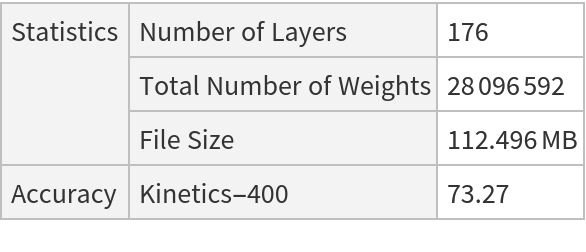## Examples

### Resource retrieval

Get the pre-trained net:

 In:=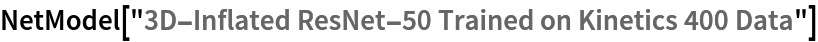Out=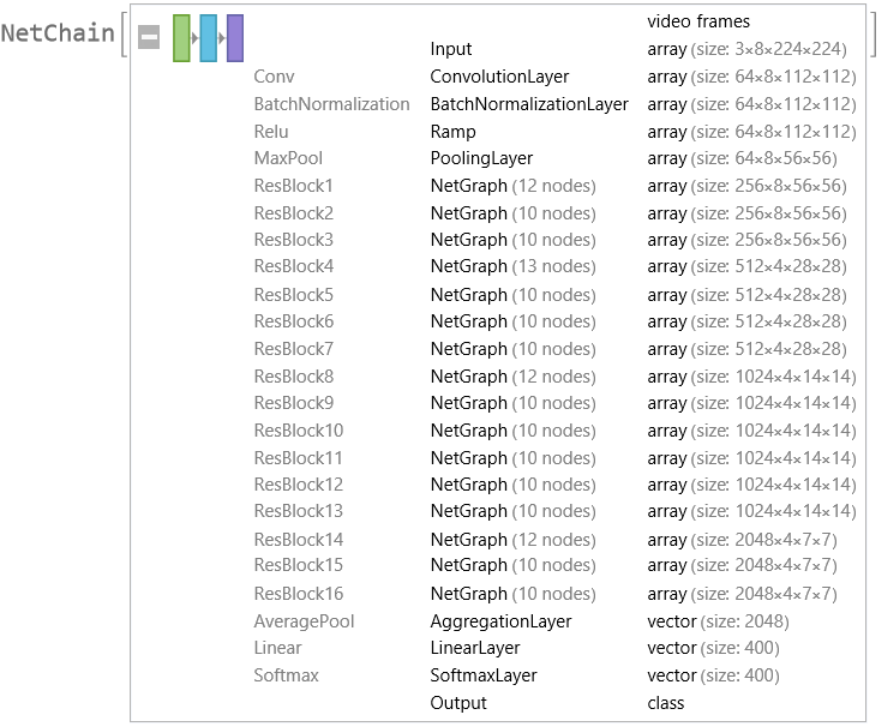### Basic usage

Classify a video:

 In:=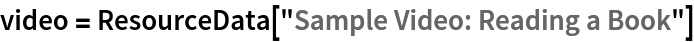Out=In:=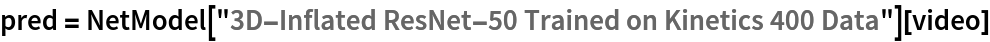Out=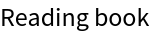Obtain the probabilities predicted by the net:

 In:=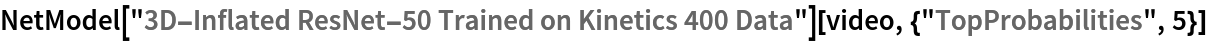Out=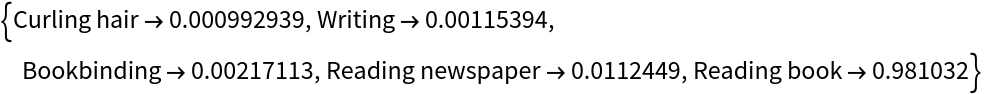### Feature extraction

Remove the last two layers of the trained net so that the net produces a vector representation of an image:

 In:=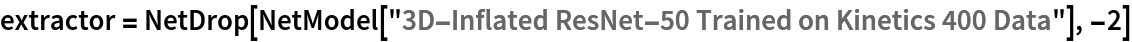Out=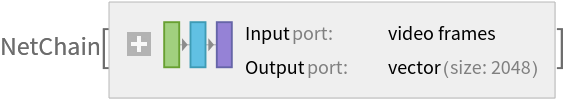Get a set of videos:

 In:=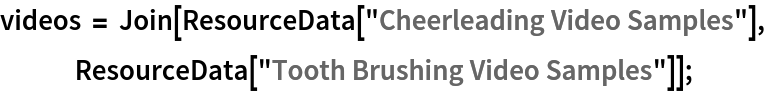Visualize the features of a set of videos:

 In:=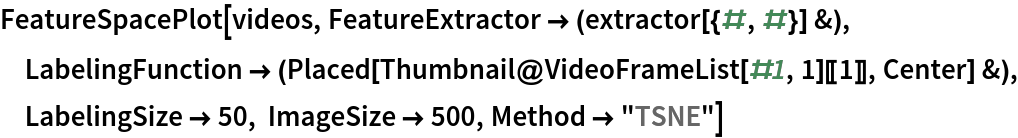Out=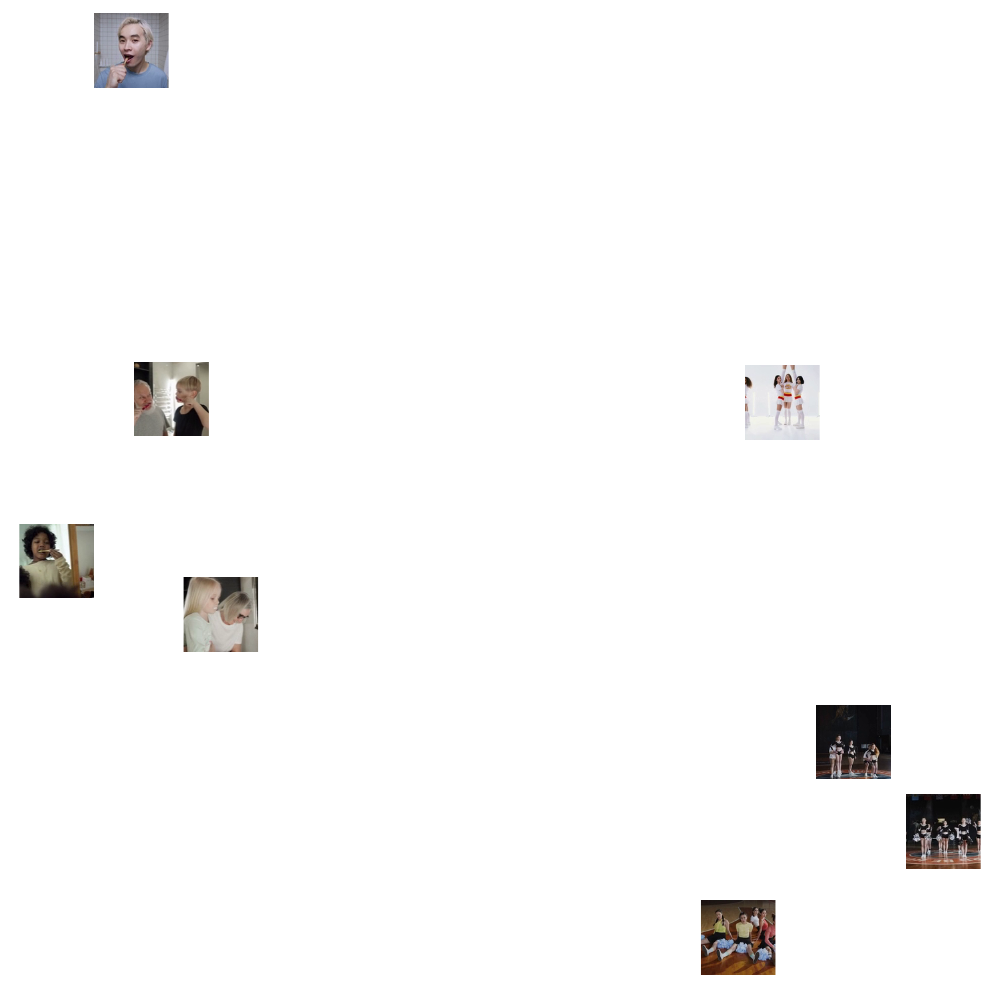### Transfer learning

Use the pre-trained model to build a classifier for telling apart images from two action classes not present in the dataset. Create a test set and a training set:

 In:=In:=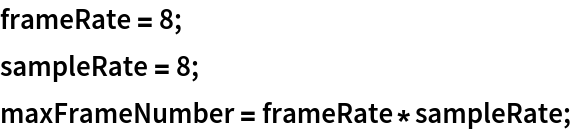In:=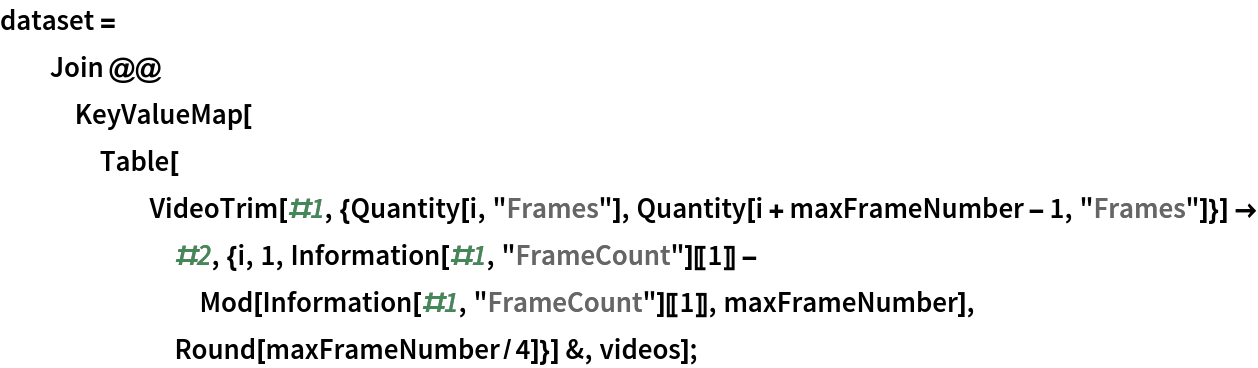In:=Remove the last two layers from the pre-trained net:

 In:=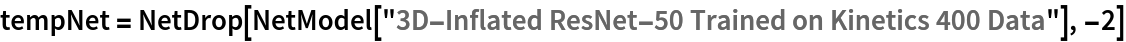Out=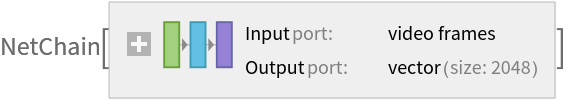Create a new net composed of the pre-trained net followed by a linear layer, an aggregation layer and a softmax layer:

 In:=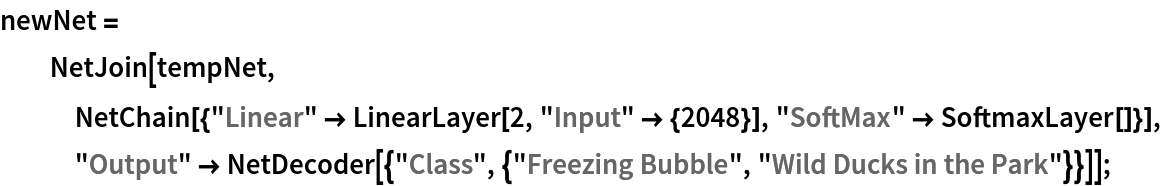Train on the dataset, freezing all the weights except for those in the "Linear" layer (use TargetDevice -> "GPU" for training on a GPU):

 In:=Out=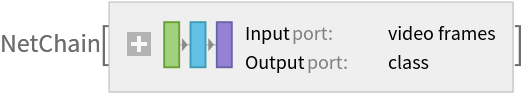Perfect accuracy is obtained on the test set:

 In:=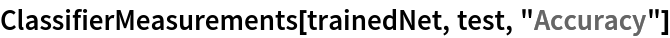Out=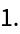### Net information

Inspect the number of parameters of all arrays in the net:

 In:=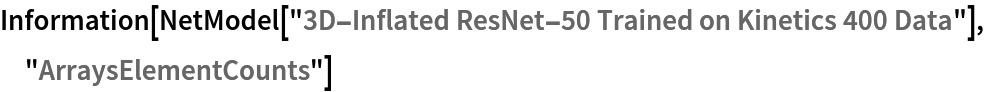Out=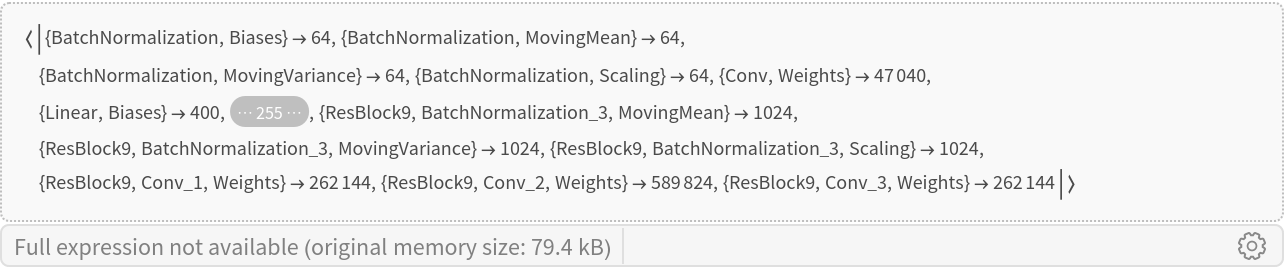Obtain the total number of parameters:

 In:=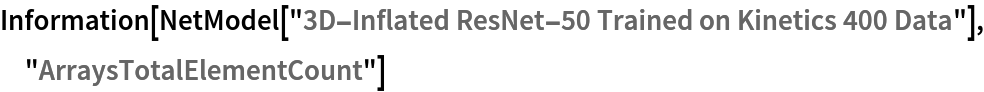Out=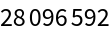Obtain the layer type counts:

 In:=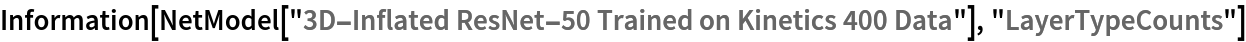Out=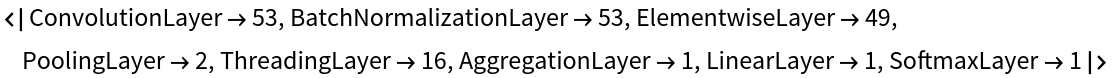Display the summary graphic:

 In:=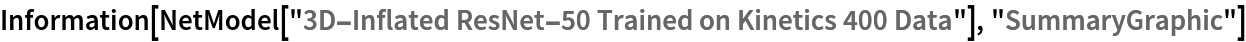Out=